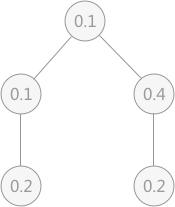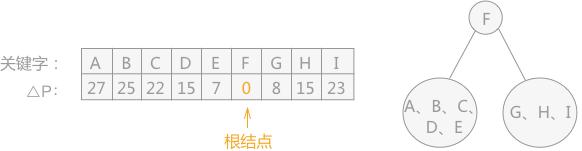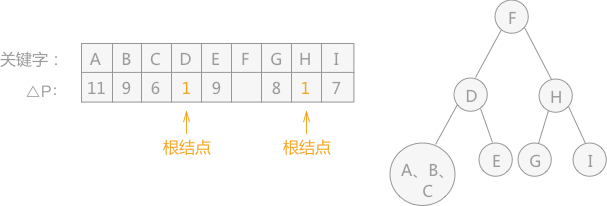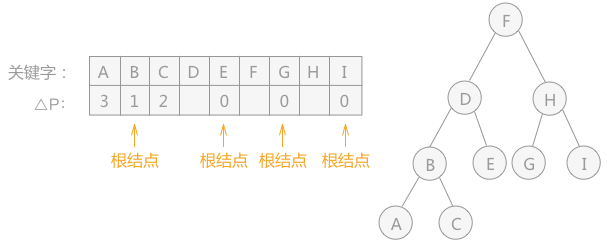## 算法思想

### 算法思想例子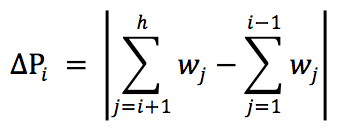ASL = 判定树中的各节点的查找概率 * 所在层次

ASL=0.41 + 0.22 + 0.22 + 0.13 + 0.1*3=1.8

## 次优查找树的构建方法

### 构建二叉树方式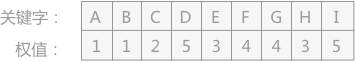### 算法实现

 `````` 1 2 3 4 5 6 7 8 9 10 11 12 13 14 15 16 17 18 19 20 21 22 23 24 25 26 27 28 29 30 31 32 33 34 35 `````` ``````typedef int KeyType;//定义关键字类型 typedef struct{ KeyType key; }ElemType;//定义元素类型 typedef struct BiTNode{ ElemType data; struct BiTNode *lchild, *rchild; }BiTNode, *BiTree; //定义变量 int i; int min; int dw; //创建次优查找树，R数组为查找表，sw数组为存储的各关键字的概率（权值），low和high表示的sw数组中的权值的范围 void SecondOptimal(BiTree T, ElemType R[], float sw[], int low, int high){ //由有序表R[low...high]及其累计权值表sw（其中sw==0）递归构造次优查找树 i = low; min = abs(sw[high] - sw[low]); dw = sw[high] + sw[low - 1]; //选择最小的△Pi值 for (int j = low+1; j <=high; j++){ if (abs(dw-sw[j]-sw[j-1])data = R[i];//生成结点（第一次生成根） if (i == low) T->lchild = NULL;//左子树空 else SecondOptimal(T->lchild, R, sw, low, i - 1);//构造左子树 if (i == high) T->rchild = NULL;//右子树空 else SecondOptimal(T->rchild, R, sw, i + 1, high);//构造右子树 } ``````

### 完整实例演示